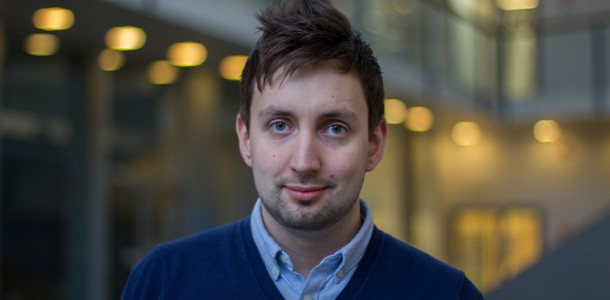###### The extended supporting hyperplane algorithm and applications

April 25, 2014

I joined the OSE group at the beginning of 2014, after I received my master’s degree in chemical engineering from Åbo Akademi University. I’m currently working on a new algorithm for solving convex and pseudo convex locally Lipschitz continuous mixed-integer nonlinear programming (MINLP) problems.

The algorithm is loosely based on the extended cutting plane (ECP) algorithm, however instead of utilizing cutting planes supporting hyper planes are generated . The algorithm also uses two preprocessing steps to rapidly generate supporting hyper planes. The first preprocessing step solves integer relaxed linear programming (LP) sub problems and one dimensional sub problems to generate supporting hyper planes. These sub problems are significantly simpler than the original problem and can be solved rapidly. The preprocessing steps will efficiently give a tight linear approximation of the feasible region of the MINLP problem and therefore it should only require a few mixed-integer linear programming (MILP) sub problems to find the global optimum of the MINLP problem. Therefore this algorithm should be significantly faster than the ECP algorithm.

Solving convex MINLP problems to global optimum efficiently is still difficult in some cases, even if there are several algorithms available. The stability and efficiency of MINLP solvers is of paramount importance especially when utilized in real-world applications. Global solution techniques for non-convex MINLP problems may also require convex MINLP sub solvers [2, 3].

Some references
 T. Westerlund and F. Pettersson. An extended cutting plane method for solving convex MINLP problems.
Computers & Chemical Engineering, 19:131–136, 1995.

 C.A. Floudas and C.E. Gounaris. A review of recent advances in global optimization. Journal of Global Optimization, 45(1):3–38, 2009.

 A. Lundell, A. Skjäl, and T. Westerlund. A reformulation framework for global optimization. Journal ofGlobal Optimization, 57(1):115–141, 2013.